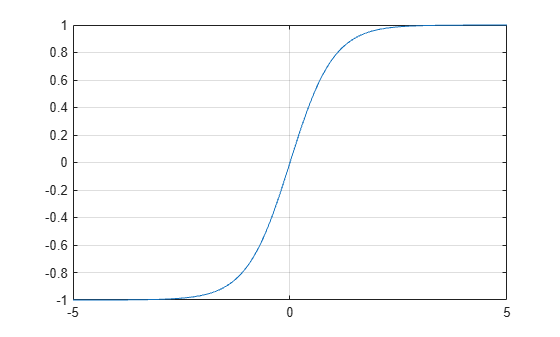# tanh

Hyperbolic tangent

## Syntax

``Y = tanh(X)``

## Description

example

````Y = tanh(X)` returns the hyperbolic tangent of the elements of `X`. The `tanh` function operates element-wise on arrays. The function accepts both real and complex inputs. All angles are in radians.```

## Examples

collapse all

Create a vector and calculate the hyperbolic tangent of each value.

```X = [0 pi 2*pi 3*pi]; Y = tanh(X)```
```Y = 1×4 0 0.9963 1.0000 1.0000 ```

Plot the hyperbolic tangent function over the domain $-5\le x\le 5$.

```x = -5:0.01:5; y = tanh(x); plot(x,y) grid on```## Input Arguments

collapse all

Input angles in radians, specified as a scalar, vector, matrix, or multidimensional array.

Data Types: `single` | `double`
Complex Number Support: Yes

collapse all

### Hyperbolic Tangent

The hyperbolic tangent of an angle x is the ratio of the hyperbolic sine and hyperbolic cosine

`$\mathrm{tanh}\left(x\right)=\frac{\mathrm{sinh}\left(x\right)}{\mathrm{cosh}\left(x\right)}=\frac{{e}^{2x}-1}{{e}^{2x}+1}.$`

In terms of the traditional tangent function with a complex argument, the identity is

`$\mathrm{tanh}\left(x\right)=-i\mathrm{tan}\left(ix\right)\text{\hspace{0.17em}}.$`